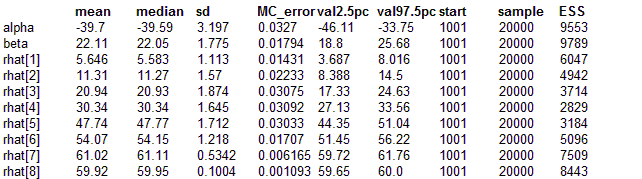#### Beetles: choice of link function

Dobson (1983) analyses binary dose-response data published by Bliss (1935), in which the numbers of beetles killed after 5 hour exposure to carbon disulphide at N = 8 different concentrations are recorded: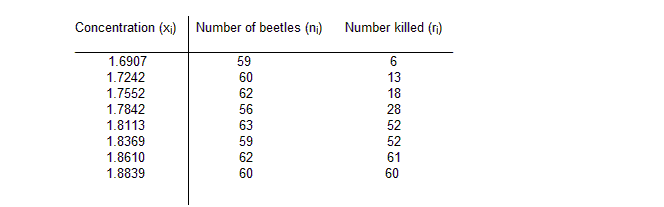We assume that the observed number of deaths ri at each concentration xi is binomial with sample size ni and true rate pi. Plausible models for pi include the logistic, probit and extreme value (complimentary log-log) models, as follows
pi = exp(α + βxi) / (1 + exp(α + βxi)

pi = Phi(α + βxi)

pi = 1 - exp(-exp(α + βxi))

The corresponding graph is shown below: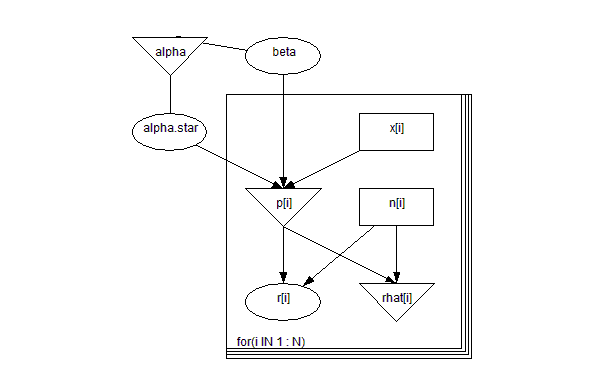model
{
for( i in 1 : N ) {
r[i] ~ dbin(p[i],n[i])
logit(p[i]) <- alpha.star + beta * (x[i] - mean(x[]))
rhat[i] <- n[i] * p[i]
}
alpha <- alpha.star - beta * mean(x[])
beta ~ dnorm(0.0,0.001)
alpha.star ~ dnorm(0.0,0.001)
}

##### Data
``` list( x = c(1.6907, 1.7242, 1.7552, 1.7842, 1.8113, 1.8369, 1.8610, 1.8839),      n = c(59, 60, 62, 56, 63, 59, 62, 60),      r = c(6, 13, 18, 28, 52, 53, 61, 60), N = 8) ```
##### Inits for chain 1
``` list(alpha.star=0, beta=0) ```

##### Inits for chain 2
``` list(alpha.star=1, beta=1) ```
Results
Logit model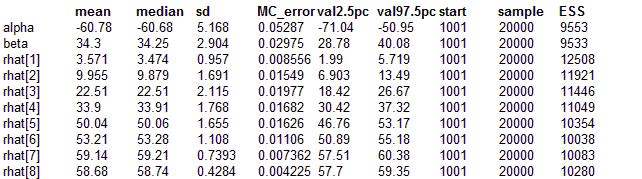Probit model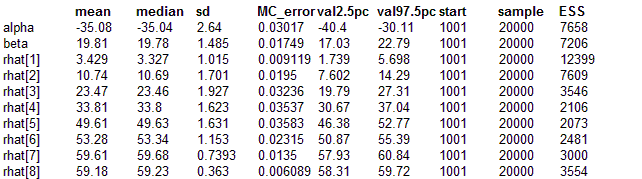Extreme value (cloglog) model